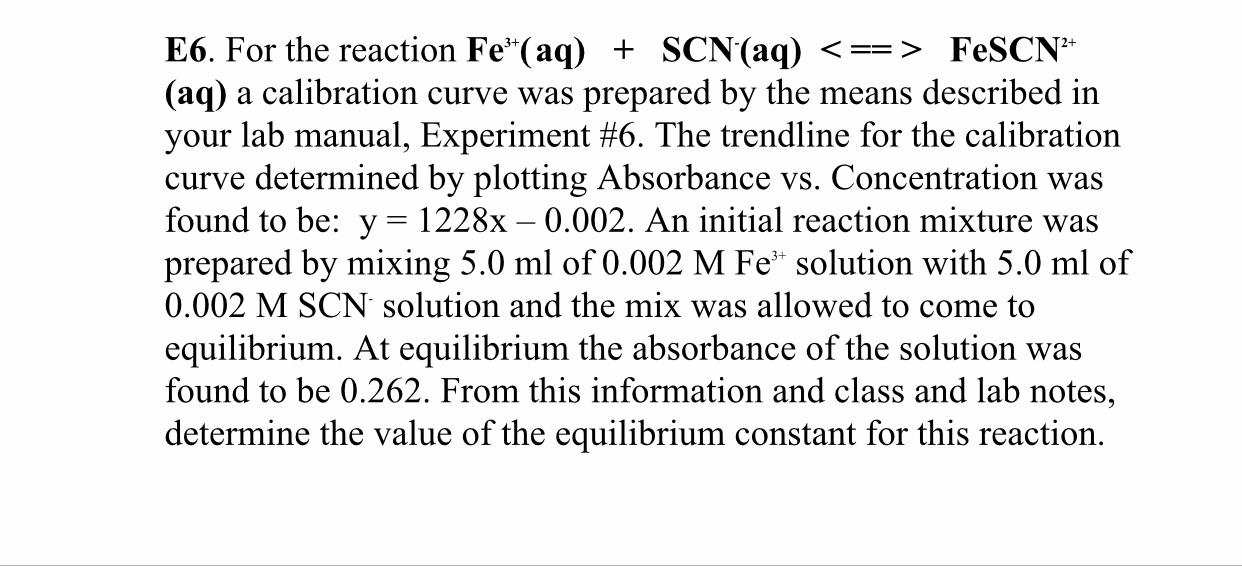# For the reaction Fe^3+ (aq) + SCN^- (aq) ⇌ FeSCN^2+ (aq) a calibration curve was prepared by the means described in your lab manual, Experiment #6. The trendline for the calibration curve determined by plotting Absorbance vs Concentration was found to be: y = 1228x - 0.002. An initial reaction mixture was prepared by mixing 5.0 mL of 0.002 M Fe^3+ solution with 5.0 mL of 0.002 M SCN^- solution and the mix was allowed to come to equilibrium. At equilibrium the absorbance of the solution was found to be 0.262. From this information and class and lab notes, determine the value of the equilibrium constant for this reaction.